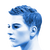1. Home
2. >
3. Why is Math.max() smaller than Math.min()?3

# Why is Math.max() smaller than Math.min()?shantanu singh

The fact that Math.max() > Math.min() returns false sounds wrong, but it actually makes a lot of sense.

If no arguments are given, Math.min() returns infinity and Math.max() returns -infinity . This is simply part of the specification for the max() and min() methods, but there is good logic behind the choice (Code given below)

If -infinity was considered the default argument of Math.min() , then every result would be -infinity. Whereas, if the default argument is infinity , the addition of any other argument would return that number — and that’s the behaviour we want.

# javascript
```Math.min(1)
// 1
Math.min(1, infinity)
// 1
Math.min(1, -infinity)
// -infinity```

### Discussions (1)

Math.max() starts with the search of -infinity whereas Math.min() starts with the search of Infinity

• 1